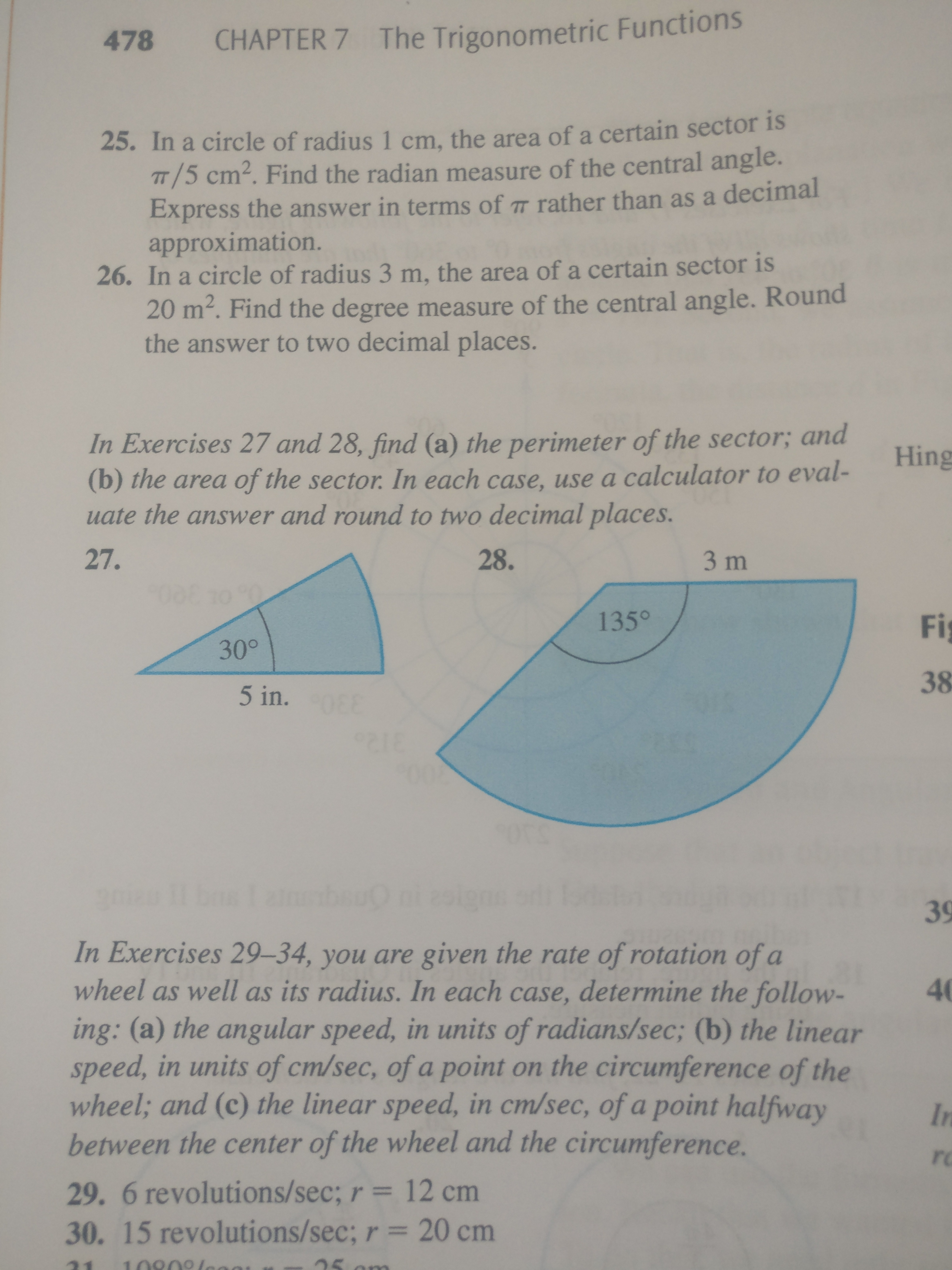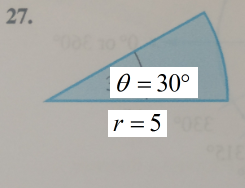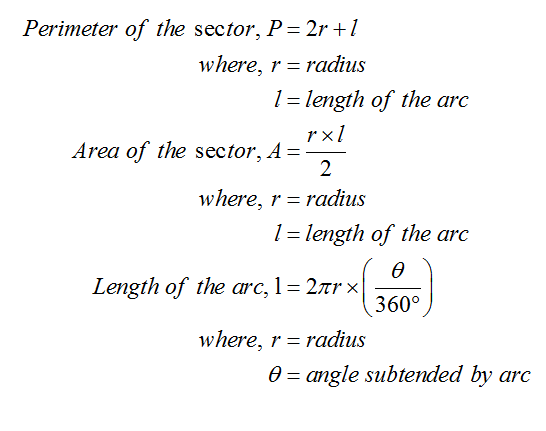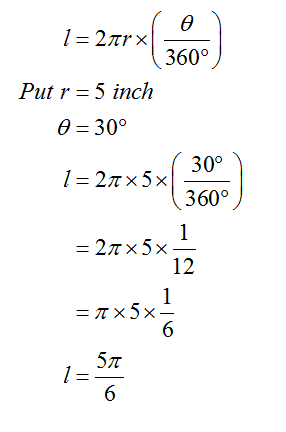# 478CHAPTER 7 The Trigonometric Functions25. In a circle of radius 1 cm, the area of a certain sector isTT/5 cm². Find the radian measure of the central angle.Express the answer in terms of 7 rather than as a decimalapproximation.26. In a circle of radius 3 m, the area of a certain sector is20 m². Find the degree measure of the central angle. Roundthe answer to two decimal places.In Exercises 27 and 28, find (a) the perimeter of the sector; andHing(b) the area of the sector. In each case, use a calculator to eval-uate the answer and round to two decimal places.27.28.3 m135°Fi30°385 in.0039In Exercises 29–34, you are given the rate of rotation of awheel as well as its radius. In each case, determine the follow-40ing: (a) the angular speed, in units of radians/sec; (b) the linearspeed, in units of cm/sec, of a point on the circumference of thewheel; and (c) the linear speed, in cm/sec, of a point halfwaybetween the center of the wheel and the circumference.Inro29. 6 revolutions/sec; r = 12 cm30. 15 revolutions/sec; r = 20 cm10909lene

Question
1 views

Number 27help_outlineImage Transcriptionclose478 CHAPTER 7 The Trigonometric Functions 25. In a circle of radius 1 cm, the area of a certain sector is TT/5 cm². Find the radian measure of the central angle. Express the answer in terms of 7 rather than as a decimal approximation. 26. In a circle of radius 3 m, the area of a certain sector is 20 m². Find the degree measure of the central angle. Round the answer to two decimal places. In Exercises 27 and 28, find (a) the perimeter of the sector; and Hing (b) the area of the sector. In each case, use a calculator to eval- uate the answer and round to two decimal places. 27. 28. 3 m 135° Fi 30° 38 5 in. 00 39 In Exercises 29–34, you are given the rate of rotation of a wheel as well as its radius. In each case, determine the follow- 40 ing: (a) the angular speed, in units of radians/sec; (b) the linear speed, in units of cm/sec, of a point on the circumference of the wheel; and (c) the linear speed, in cm/sec, of a point halfway between the center of the wheel and the circumference. In ro 29. 6 revolutions/sec; r = 12 cm 30. 15 revolutions/sec; r = 20 cm 10909lene fullscreen
check_circle

Step 1

Consider the given diagram for 27.Step 2

Consider the formula to calculate the perimeter, area for the sector of a circle and the length for the length of the arc.Step 3

Now, calculate the arc length....

### Want to see the full answer?

See Solution

#### Want to see this answer and more?

Solutions are written by subject experts who are available 24/7. Questions are typically answered within 1 hour.*

See Solution
*Response times may vary by subject and question.
Tagged in